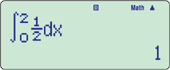# fx-570ES PLUS

Close

• The colors may differ slightly from the original.

## fx-570ES PLUS

Number of Functions : 417
Natural textbook display
Display expression same as textbook.
AAA × 1 (R03)

## Features

### New functions

• New equation mode
• Random integers

### Standard functions

• Fraction calculations
• Combination and permutation
• Statistics (List-based STAT data editor, standard deviation, regression analysis)
• 9 variables
• Table function
• Comes with new slide-on hard case

### fx-82ES PLUS/85ES PLUS/350ES PLUS functions, in addition to:

• Equation calculations
• Integration/differential calculations
• Matrix calculations
• Vector calculations
• Complex number calculations
• CALC function
• SOLVE function
• Base-n calculation

#### Integration#### Complex number calculations#### Differential#### Vector#### Matrix operations## Specifications

• Non Programmable

•Number of Functions : 417

•Natural textbook display Display expression same as textbook.

•AAA × 1 (R03)

•10 + 2 digits 10-digit mantissa + 2-digit exponential display.

•Dot matrix display High-resolution screen provides beautiful looking graphs every time.

•Multi-replay Quick and easy recall of previously executed formulas for editing and re-execution.

•Plastic keys Designed and engineered for easy operation.

• Basic Statistics Standard statistics functions such as Mean, SUM, Standard Deviation, and Regression

• Basic Mathematical Functions Trigonometric, Exponential logarithmic, etc.

• Table Generation You can create a numerical table based on that expression by registering a function expression. In addition, graph functions can be represented by graphs.

• Scientific Constants You can use constant symbols used in physics, science classes.

• Vector

•List based STAT-data editor Viewing and editing of input data in list format, showing data groups (x-data, y-data, frequency) and surrounding data.

• AAA-size Battery

• Differentiation

• Non Graphing

• Matrix

• Equation

• Integration

•Metric conversion function You can convert a number in one unit to a number in a different unit.

• Complex number calculation